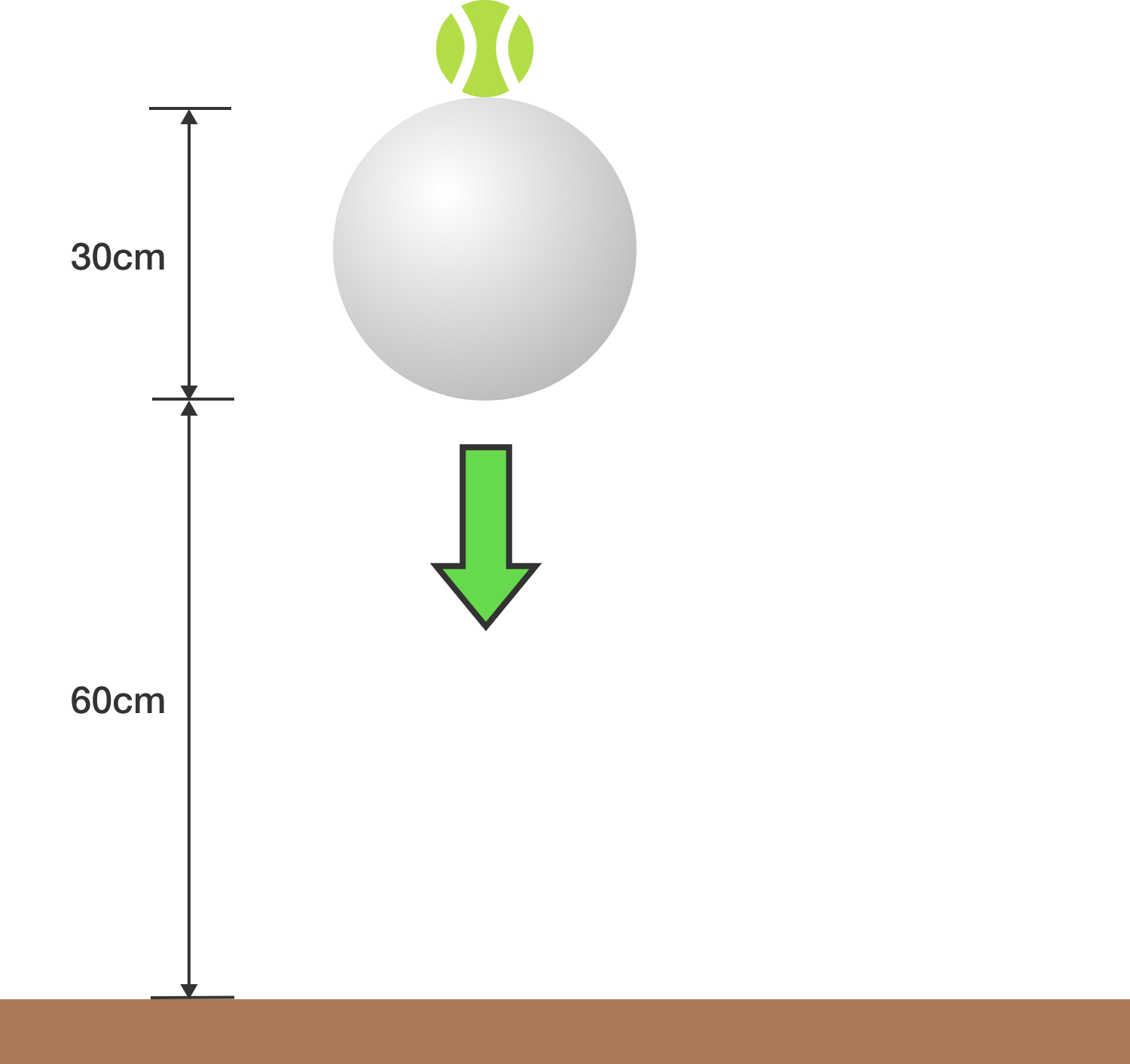# Bouncing ballsA tennis ball rests directly on top of a cannon ball, which has a much larger mass, i.e. $M_\textrm{cannonball} \gg M_\textrm{tennis}.$

When the balls are dropped, to what height (in $\si{\centi\meter}$) will the bottom of the tennis ball bounce?


Details and Assumptions:

• The bottom of the cannon ball starts at a height of $h = \SI{60}{\centi\meter}$ from the ground, and the cannon ball has diameter $d=\SI{30}{\centi\meter}$.
• All collisions are perfectly elastic, and wind resistance is ignored.
×

Problem Loading...

Note Loading...

Set Loading...